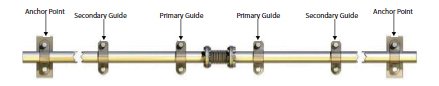## Pipe Anchors for AX1, AX2 and AX3 Expansion Compensators

Pipe anchors are needed to overcome the forces set up when axial bellows and under pressure. The following conditions can exist:

Pipeline under pressure test.

Pipeline under working pressure and temperature.

Each condition must be investigated:

• Pipeline under test pressure – this force is made up of test pressure multiplied by the effective area.
• Pipeline under working pressure – the force is made up of three components;
1. The force to overcome internal pressure; i.e working pressure multiplied by effective area.
2. The force to compress the expansion joint. This is calculated by using the force to compress in Newtons/mm.
3. The force to overcome the friction of pipe movement, which can be estimated at 30N per metre of pipeline for each 25mm of pipe diameter. This assumes about the worst possible condition of face to face sliding supports. Coefficient of friction about 0.4. If hangers or rollers are used, reduce the figure to 15N per metre of pipeline.

Thus the Total Force = a + b + c

Expansion joints should not be removed during a pressure test. The purpose of a pressure test is to put the pipeline under stresses greater than those which occur under working conditions. If the expansion joints are removed, the anchors and guides are not subjected to any stresses and therefore faults in their design will not show up.

When positioning anchors, great care should be taken to ensure that the main structure to which the anchor is attached, is in fact strong enough to withstand the forces transmitted by the anchor. Also ensure that when a number of pipes are anchored at one point, the total force under working conditions is considered.

Below is an example showing the anchor forces set up under different conditions.

In these examples 1 bar = 100kN/m².Calculate the anchor force for a 25m long pipeline of 32mm NB at 6 bar working pressure and temperature of 82°C, using modular slide guides.

At 82°C the expansion in mm/metre is 1.0. Therefore the total expansion is 1.0 x 25 = 25mm.

Test pressure is 1.5 x working pressure = 1.5 x 6 = 9 bar (=900 kN/m²).

Effective area can be taken from the product catalogue, in this case 16cm².

For a pipeline under the test conditions, TOTAL FORCE = test pressure x effective area = 900 x 16 x 0.1 = 1,440N. Working Pressure of 6 (=600kN/m²).

a = 600 x 16 x 0.1 = 960N

b = 15 x 25 = 375N

c = 15 x 25 x (32/25) = 480N

Therefore, TOTAL FORCE = 960 + 375 + 480 = 1,815N